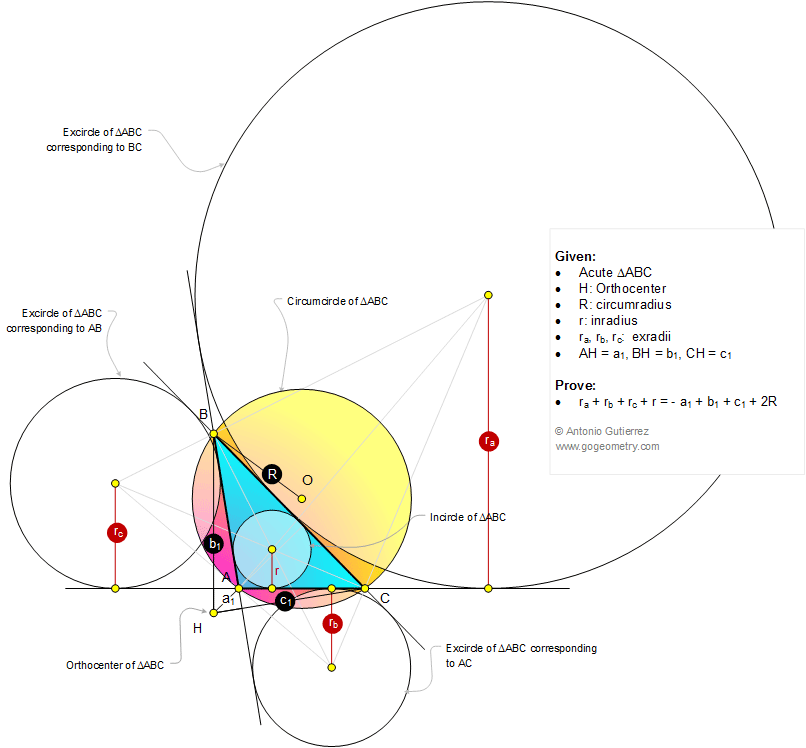Infographic Geometry Problem 1068: Obtuse Triangle, Orthocenter, Circumradius, Inradius, Exradii, Distance, Diameter. Level: High School, SAT Prep, College, Mathematics Education

< PREVIOUS PROBLEM  |  NEXT PROBLEM >

 The figure shows a triangle ABC with obtuse angle A, the orthocenter H, the circumradius R, the inradius r, and the exradii, ra, rb, and rc. If AH = a1, BH = b1, and CH = c1, prove that ra + rb + rc + r = - a1 + b1 + c1 + 2R.Home | Search | Geometry | Problems | All Problems | Open Problems | Visual Index | 10 Problems | 1061-1070 | Triangle | Orthocenter | Altitude | Circle | Circumradius | Inradius | Exradius | Email | by Antonio Gutierrez Add a solution to the problem 1068 Last updated: Dec 21, 2014